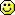# Parallelism using Bitmaps

If you think about it this way you can combine setvl, and predication, and indeed vector length, by always working with bitmaps.

So: you have 32 WARL CSRs , called X0, ... X31 (or perhaps 2 banks of 32 CSR's and have a set of additional CSR's FX0,... FX31)

Each contains a bitmap of length 32 (assuming we only have the standard registers)

By default, X0 contains 1<<0, X1 contains 1<<1, X2 contains 1 << 2, ...

now an instruction like

``````add x1 x2 x3
``````

is reinterpreted as referring to the CSR's rather than individual registers. i.e. under simple V it means

``````add X1, X2, X3
``````

and it has the following semantics:

``````let rds   = registers in bitmap X1
let rs1s = registers in bitmap X2 repeated periodically in order of register number to the length of X1
let rs2s =  registers in bitmap X3 repeated periodically in order of register number to the length of X1

parallelfor (rd, rs1, rs2)  in  (rds[i],rs1s[i], rs2s[i]) where i = 0 to length(rds) - 1
``````

example:

``````X1 <- 0b011111
X2 <- 0b1011
X3 <-  0b00010

> Anyways my point was, for me it would have been more intuitive
> and easier to grasp if it showed:
> X1 -> b011111 (meaning x4,x3,x2,x1,x0)
> X2 -> b001011 (meaning x3,x1,x0)
> X3 -> b000010 (meaning x1)
``````

then

``````rd1s = [x1, x2, x3, x4, x5]
rs1s = [x0, x2, x3, x0, x2]
rs2s = [x3, x3, x3, x3, x3]
``````

and

``````add X1, X2, X3
``````

is interpreted as

``````parallel{
add x4, x0, x3   # x2 and x3 have their original values!
add x5, x2, x3   # x2 and x3 have their original values!
}
``````

This means that the analogue of setvl is simply the "write any" of setting the bitmap, and the analogue of the return value of setvl, is the "read legal" of the CSR. Moreover popc would tell you how many operations are scheduled in parallel so you know how often you have to repeat a sequential loop.

Notes:

Thinking about it more, a bitset for X0 seems a bad idea, or equivalently X0 should be the immutable  bitset {x0}. That suggests FX0, ... FX31 is a good idea.

what would it mean, to do ops with x0?  it would mean "always add 0" and so on.  it sounds kinda useful.  like MV being add r1, r2, x0.  it would completely pointless to have anything other than "all 1s" in it though i think# pseudocode for decoding ops

``````uint32 XB;   // global, assume RV32 for now: CSRs for bitmapping
uint32 regs; // global, actual (integer) register file

// gets current ACTUAL register to be used
// XB had better not be empty...
int regdecode(int rn, int *offs)
{
int bmap = XB[rn];
int _offs = *offs;
while (1)
{
int _newoffs = (_offs + 1) & 0x1f; // 32 regs, modulo
if (bmap & (1<<_offs))
{
*offs = _newoffs;
return _offs;
}
_offs = _newoffs;
}
}
``````

``````op_add(int rd, int rs1, int rs2)
{
int id=0, irs1=0, irs2=0;
int VL = pcnt(XB[rd];
for (int i = 0; i < VL; i++)
{
int actualrd  = regdecode(rd , &id);
int actualrs1 = regdecode(rs1, &irs1);
int actualrs2 = regdecode(rs2, &irs2);
regs[actualrd] = regs[actualrs1] + regs[actualrs2];
}
}
``````